# Simple Interest - Quantitative Aptitude (MCQ) questions

Dear Readers, Welcome to Quantitative Aptitude Simple Interest questions and answers with explanation. These Simple Interest solved examples with shortcuts and tricks will help you learn and practice for your Placement Test and competitive exams like Bank PO, IBPS PO, SBI PO, RRB PO, RBI Assistant, LIC,SSC, MBA - MAT, XAT, CAT, NMAT, UPSC, NET etc.

After practicing these tricky Simple Interest multiple choice questions, you will be exam ready to deal with any objective type questions.

1)   Sam borrowed some money from his friend at simple interest of 6% per annum. He returned his friend Rs. 15600. After how much time did Sam return the money if he borrowed Rs. 12000?
- Published on 03 May 17

a. 8 years
b. 2.5 years
c. 5 years
d. 3.5 years
 Answer  Explanation ANSWER: 5 years Explanation: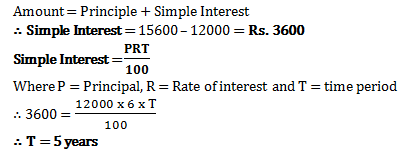2)   Kabir paid Rs. 9600 as interest on a loan he took 5 years ago at 16% rate of simple interest. What was the amount he took as loan?
- Published on 03 May 17

a. Rs. 16400
b. Rs. 12000
c. Rs. 12500
d. Rs. 18000
 Answer  Explanation ANSWER: Rs. 12000 Explanation: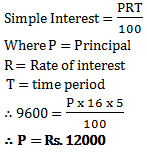3)   Suresh for 2 years invested Rs. 500 in SBI. He also invested Rs. 300 in ICICI for 4 years. At the end he received Rs. 220 from both banks as simple interest. What must have been rate of interest?
- Published on 03 May 17

a. 10%
b. 12%
c. 11%
d. 5.5%
 Answer  Explanation ANSWER: 10% Explanation: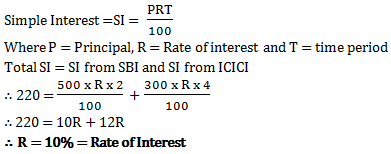4)   Raman paid Rs. 11400 as interest after 9 years. He had borrowed some money at rate of 6% for first two years, 9% for next three years and 14% for rest of the period. How much money did he borrow?
- Published on 13 Apr 17

a. Rs. 10000
b. Rs. 15000
c. Rs. 12000
d. Rs. 12500
 Answer  Explanation ANSWER: Rs. 12000 Explanation: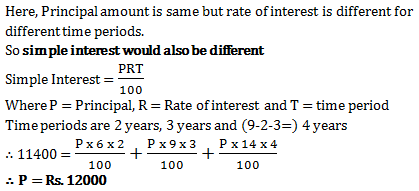5)   Ram gets Rs. 2600 for Rs. 2000 in 5 years at some rate of simple interest. Had he invested in other places where rate of simple interest is 3% more than current rate, how much would have Ram got in same time?
- Published on 13 Apr 17

a. Rs. 2900
b. Rs. 3000
c. Rs. 3100
d. Rs. 2800
 Answer  Explanation ANSWER: Rs. 2900 Explanation: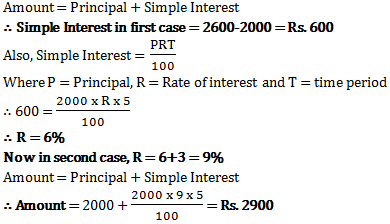6)   Guddi invested some money in a bank at rate of 6% per annum. At simple interest, after 9 years, she got Rs. 8470. How much did she invest?
- Published on 11 Apr 17

a. Rs. 5250
b. Rs. 6550
c. Rs. 6400
d. Rs. 5500
 Answer  Explanation ANSWER: Rs. 5500 Explanation: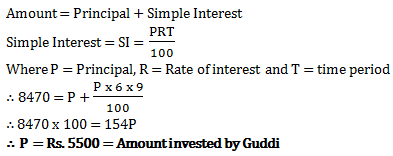7)   Aman got a salary of Rs. 8600. The salary was invested by him in two parts. Find the difference between the two parts of his salary, if in first part he got some simple interest at 15% per annum in 4 years, which was same as the second part which he invested at 20% for 3 years.
- Published on 11 Apr 17

a. Rs. 0
b. Rs. 2400
c. Rs. 100
d. Rs. 4500
 Answer  Explanation ANSWER: Rs. 0 Explanation: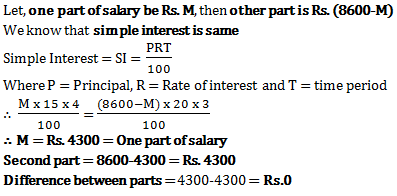8)   Aman invests Rs. 8000 at some rate of interest. Being simple interest the money doubles in 5 years. Raj sees this and invests Rs. 6250 for 3 years at same rate of interest. How much interest does Raj get?
- Published on 11 Apr 17

a. Rs. 3750
b. Rs. 6250
c. Rs. 3125
d. Rs. 4250
 Answer  Explanation ANSWER: Rs. 3750 Explanation: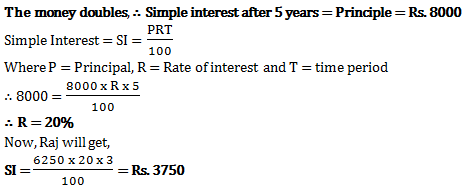9)   If simple interest for 2 years for a sum is Rs. 600 and compound interest for the same sum for 2 years and same rate of interest is Rs. 645, what will be the rate of interest?
- Published on 11 Apr 17

a. 10%
b. 15%
c. 30%
d. 5%
 Answer  Explanation ANSWER: 15% Explanation: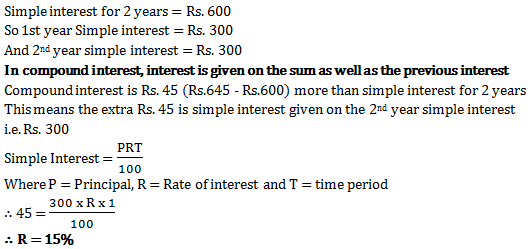10)   In 4 years the simple interest on certain sum of money is 9/25 of the principal. The annual rate of interest is
- Published on 05 Apr 17

a. 4%
b. 4(1/2)%
c. 9%
d. 10%
 Answer  Explanation ANSWER: 9% Explanation: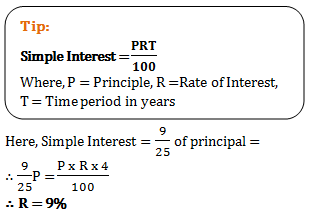1 2 3